## Summary and Analysis of Extension Program Evaluation in R

Salvatore S. Mangiafico

# Introduction to Parametric Tests

### Packages used in this chapter

The packages used in this chapter include:

•  psych

•  rcompanion

The following commands will install these packages if they are not already installed:

if(!require(psych)){install.packages("psych")}
if(!require(rcompanion)){install.packages("rcompanion")}

### When to use parametric tests

Parametric statistical tests are among the most common you’ll encounter.  They include t-test, analysis of variance, and linear regression.

They are used when the dependent variable is an interval/ratio data variable.  This might include variables measured in science such as fish length, child height, crop yield weight, or pollutant concentration in water.

One advantage of using parametric statistical tests is that your audience will likely be familiar with the techniques and interpretation of the results.  These tests are also often more flexible and more powerful than their nonparametric analogues.

Their major drawback is that all parametric tests assume something about the distribution of the underlying data.  If these assumptions are violated, the resultant test statistics will not be valid, and the tests will not be as powerful as for cases when assumptions are met.

#### Count data may not be appropriate for common parametric tests

A frequent error is to use common parametric models and tests with count data for the dependent variable.  Instead, count data could be analyzed either by using tests for nominal data or by using regression methods appropriate for count data.  These include Poisson regression, negative binomial regression, and zero-inflated Poisson regression.  See the Regression for Count Data chapter.

##### When to use parametric tests for count data

It is sometimes permissible to use common parametric tests for count data or other discrete data.  They can be used in cases where counts are used as a type of measurement of some property of subjects, provided that 1) the distribution of data or residuals from the analysis approximately meet test assumptions; and 2) there are few or no counts at or close to zero, or close to a maximum, if one exists.  Permissible examples might include test scores, age, or number of steps taken during the day.  Technically, each of these measurements is bound by zero, and are discrete rather than continuous measurements.  However, if other conditions are met, it is reasonable to handle them as if they were continuous measurement variables.

This kind of count data will sometimes need to be transformed to meet the assumptions of parametric analysis.  Square root and logarithmic transformations are common.  However, if there are many counts at or near zero, transformation is unlikely to help.  It is usually not worth the effort to attempt to force count data to meet the assumptions of parametric analysis with transformations, since there are more appropriate methods available.

#### Percentage and proportion data

Percentage and proportion data are often inappropriate for parametric statistics for the same reasons given for count data.  They will often not meet the assumptions of the tests, and are particularly problematic if there are some or many observations close to 0 or 1.  The arcsine transformation was used traditionally, but logistic regression or beta regression may be more appropriate. However, if assumptions are approximately met, parametric analyses could be used.

### Assumptions in parametric statistics

All parametric analyses have assumptions about the underlying data, and these assumptions should be confirmed or assumed with good reason when using these tests.  If these assumptions are violated, the resulting statistics and conclusions will not be valid, and the tests may lack power relative to alternative tests.

The assumptions vary among tests, but a general discussion follows.

For examples in this section, we’ll revisit the Catbus data.

Input = ("
Student  Sex     Teacher  Steps  Rating
a        female  Catbus    8000   7
b        female  Catbus    9000  10
c        female  Catbus   10000   9
d        female  Catbus    7000   5
e        female  Catbus    6000   4
f        female  Catbus    8000   8
g        male    Catbus    7000   6
h        male    Catbus    5000   5
i        male    Catbus    9000  10
j        male    Catbus    7000   8
k        female  Satsuki   8000   7
l        female  Satsuki   9000   8
m        female  Satsuki   9000   8
n        female  Satsuki   8000   9
o        male    Satsuki   6000   5
p        male    Satsuki   8000   9
q        male    Satsuki   7000   6
r        female  Totoro   10000  10
s        female  Totoro    9000  10
t        female  Totoro    8000   8
u        female  Totoro    8000   7
v        female  Totoro    6000   7
w        male    Totoro    6000   8
x        male    Totoro    8000  10
y        male    Totoro    7000   7
z        male    Totoro    7000   7
")

Data

###  Check the data frame

library(psych)

str(Data)

summary(Data)

### Remove unnecessary objects

rm(Input)

#### Random sampling

All statistical tests assume that the data captured in the sample are randomly chosen from the population as a whole.  Selection bias will obviously affect the validity of the outcome of the analysis.

#### Independent observations

Tests will also assume that observations are independent of one another, except when the analysis takes non-independence into account.  One common case of non-independent observations is in repeated measures experiments, in which the same subject is observed over time.  If you were measuring test scores of students over time, you might expect students with a high test score on one date to have a high test score on subsequent dates.  In this case the observation on one date would not be independent of observations on other dates.

The independence of observation is often assumed from good experimental design.  Also, data or residuals can be plotted, for example to see if observations from one date are correlated to those for another date.

#### Normal distribution of data or residuals

Parametric tests assume that the data come from a population of known distribution.  In particular, the tests discussed in this section assume that the distribution of the data are conditionally normal in distribution.  That is, the data are normally distributed once the effects of the variables in the model are taken into account.  Practically speaking, this means that the residuals from the analysis should be normally distributed.  This will usually be assessed with a histogram of residuals, a density plot as shown below, or with quantile–quantile plot.

A select number of tests will require that data itself be normally distributed.  This will be limited to one-sample t-test, two-sample t-test, and paired t-test.  For other tests, the distribution of the residuals will be investigated.

Residuals from an analysis are also commonly called errors.  They are the difference between the observations and the value predicted by the model.  For example, if the calculated mean of a sample is 10, and one observation is 12, the residual for this observation is 2.  If another observation is 7, the residual for this observation is –3.

Be careful not to get confused about this assumption.  You may see discussion about how “data” should be normally distributed for parametric tests.  This is usually wrong-headed.  The t-test assumes that the observations for each group are normally distributed, but if there is a difference in the groups, we might expect a bi-modal distribution, not a simple normal distribution, for the combined data.  This is why in most cases we look at the distribution of the residuals, not the raw data.

##### Optional: Considering the distributions of data for groups and their residuals

The following code is just to illustrate this principle, and isn’t normally used in analysis. First, the distributions of Steps by Sex in the Catbus data set are examined, and then the distribution of residuals — group means subtracted from each observation — is examined.

### Density plot of observations by sex for the Catbus data set

ggplot(Data,
aes(Steps, fill = Sex)) +
geom_density(position="dodge",
alpha = 0.6)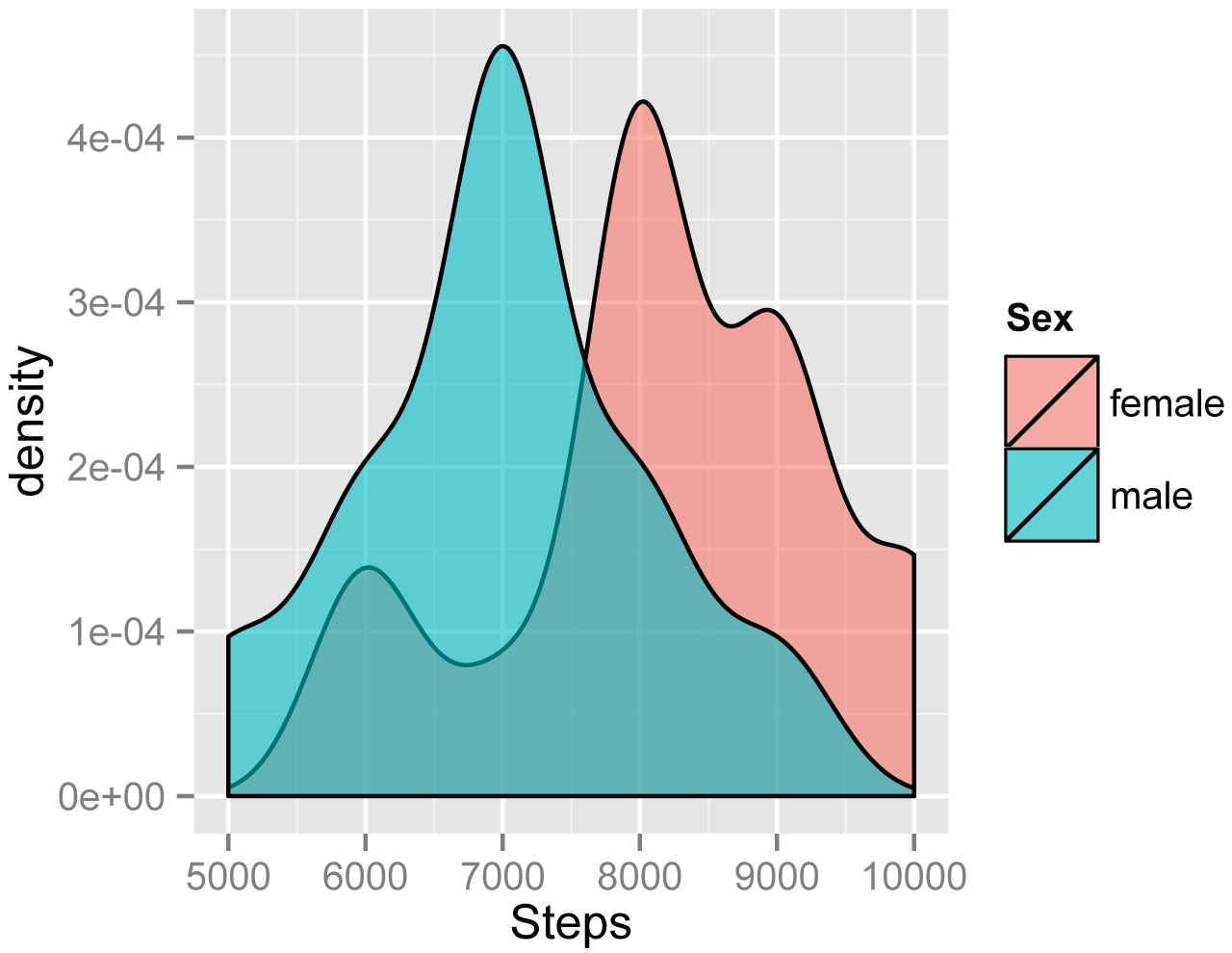The following code creates a variable Mean for Steps for each Sex in the Catbus data set, and then subtracts Steps from each mean, calling the result Residual.  This is for illustrative purposes only; you won’t normally need to manipulate data in this manner.

M1 = mean(Data\$Steps[Data\$Sex=="female"])
M2 = mean(Data\$Steps[Data\$Sex=="male"])

Data\$Mean[Data\$Sex=="female"] = M1
Data\$Mean[Data\$Sex=="male"]   = M2

Data\$Residual = Data\$Steps - Data\$Mean

### Density plot of residuals for mean for each sex for the Catbus data set

ggplot(Data,
aes(Residual)) +
geom_density(fill = "gray")#### Homogeneity of variance

Parametric analyses will also assume a homogeneity of variance among groups.  That is, for Student's t-test comparing two groups, each group should have the same variance.

A good approach to assess this assumption is to plot residuals vs. predicted values.  The residuals should have approximately the same spread across all predicted values.

Homogeneity of variance is also called homoscedasticity.  The opposite is heteroscedasticity.

### Residuals from mean for each sex vs. mean for Catbus data set

plot(jitter(Residual) ~ Mean,
data = Data)As a technical note, by default R conducts a variant of the t-test called Welch’s t-test. This test does not assume homogeneity of variance and so can be used to compare two groups with unequal variances.

Models for two-way analysis of variance and similar analyses are constructed as linear models in which the dependent variable is predicted as a linear combination of the independent variables.

A violation of this assumption is sometimes indicated when a plot of residuals versus predicted values exhibits a curved pattern.

#### Outliers

Outliers are observations whose value is far outside what is expected.  They can play havoc with parametric analyses since they affect the distribution of the data and strongly influence the mean.

There are a variety of formal tests for detecting outliers, but they will not be discussed here.  The best approach is one that looks at residuals after an analysis.  Good tools are the “Residuals vs. leverage” plot and other plots in the “Other diagnostic plots” section below.

It’s my opinion that outliers should not be removed from data unless there is a good reason, usually when a value is impossible or a measurement error of some kind is suspected.

#### Parametric tests are somewhat robust

Some parametric tests are somewhat robust to violations of certain assumptions.  For example, the t-test is reasonably robust to violations of normality for symmetric distributions, but not to samples having unequal variances (unless Welch's t-test is used).  A one-way analysis of variance is likewise reasonably robust to violations in normality.

The upshot is that model assumptions should always be checked, but you may be able to tolerate small violations in the distribution of residuals or homoscedasticity.  Large violations will make the test invalid, though.  It is important to be honest with your assessments when checking model assumptions.  It is better to transform data, change your model, use a robust method, or use a nonparametric test than to not have confidence in your analysis.

### Assessing model assumptions

For this example, we’ll revisit the Catbus data.  We’ll then define a linear model where Steps is the dependent variable and Sex and Teacher are the independent variables.

Input = ("
Student  Sex     Teacher  Steps  Rating
a        female  Catbus    8000   7
b        female  Catbus    9000  10
c        female  Catbus   10000   9
d        female  Catbus    7000   5
e        female  Catbus    6000   4
f        female  Catbus    8000   8
g        male    Catbus    7000   6
h        male    Catbus    5000   5
i        male    Catbus    9000  10
j        male    Catbus    7000   8
k        female  Satsuki   8000   7
l        female  Satsuki   9000   8
m        female  Satsuki   9000   8
n        female  Satsuki   8000   9
o        male    Satsuki   6000   5
p        male    Satsuki   8000   9
q        male    Satsuki   7000   6
r        female  Totoro   10000  10
s        female  Totoro    9000  10
t        female  Totoro    8000   8
u        female  Totoro    8000   7
v        female  Totoro    6000   7
w        male    Totoro    6000   8
x        male    Totoro    8000  10
y        male    Totoro    7000   7
z        male    Totoro    7000   7
")

###  Check the data frame

library(psych)

str(Data)

summary(Data)

### Remove unnecessary objects

rm(Input)

###### Define a linear model

model = lm(Steps ~ Sex + Teacher,
data = Data)

#### Using formal tests to assess normality of residuals

There are formal tests to assess the normality of residuals.  Common tests include Shapiro-Wilk, Anderson–Darling, Kolmogorov–Smirnov, and D’Agostino–Pearson.  These are presented in the “Optional analyses: formal tests for normality” section.

In general, I don’t recommend using these tests because their results are dependent on sample size.  When the sample size is large, the tests may indicate a statistically significant departure from normality, even if that departure is small.  And when sample sizes are small, they won’t detect departures from normality.

The article from the Fells Stats blog, in the “References” section has pretty convincing examples of these problems.

#### Skew and kurtosis

There are no definitive guidelines as to what range of skew or kurtosis are acceptable for considering residuals to be normally distributed.

In general, I would not recommend relying on skew and kurtosis calculations, but instead use histograms and other plots.

If I were forced to give advice for skewness calculations, I might say, be cautious if the absolute value is > 0.5, and consider it not normally distributed if the absolute value is > 1.0.  Some authors use 2.0 as a cutoff for normality, and others use a higher limit for kurtosis.

library(psych)

x = residuals(model)

describe(x,
type=2)        # Type of skew and kurtosis

vars  n mean      sd median trimmed     mad     min     max   range  skew kurtosis
1    1 26    0 1132.26 -39.58   11.07 1114.18 -2202.4 2123.25 4325.65 -0.13    -0.11

#### Using visual inspection to assess the normality of residuals

Usually, the best method to see if model residuals meet the assumptions of normal distribution and homoscedasticity are to plot them and inspect the plots visually.

##### Histogram with normal curve

A histogram of the residuals should be approximately normal, without excessive skew or kurtosis.  Adding a normal curve with the same mean and standard deviation as the data helps to assess the histogram.

x = residuals(model)

library(rcompanion)

normal.histogram(x)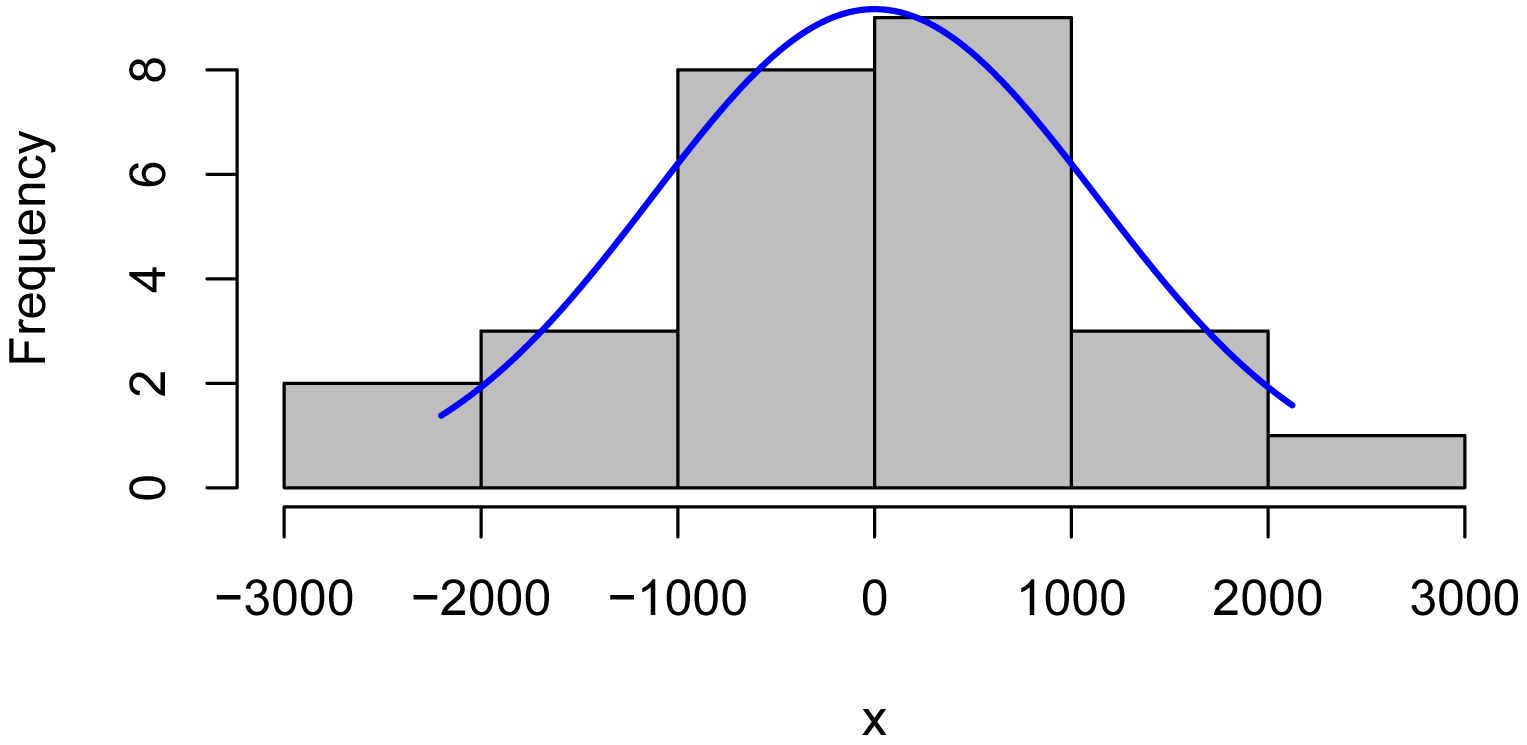##### Kernel density plot with normal curve

A kernel density plot is similar to a histogram, but is smoothed into a curve.  Sometimes a density plot gives a better representation of the distribution of data, because the appearance of the histogram depends upon how many bins are used.

The plotNormalDensity function will produce this plot.  Options include those for the plot function, as well as adjust, bw, and kernel which are passed to the density function.  col1, col2, and col3 change plot colors, and lwd changes line thickness.

library(rcompanion)

x = residuals(model)

plotNormalDensity(x,
adjust = 1)  ### Decrease this number
###  to make line less smooth##### Plot of residuals vs. fitted values

Patterns in the plot of residuals versus fitted values can indicate a lack of homoscedasticity or that errors are not independent of fitted values.

plot(fitted(model),
residuals(model))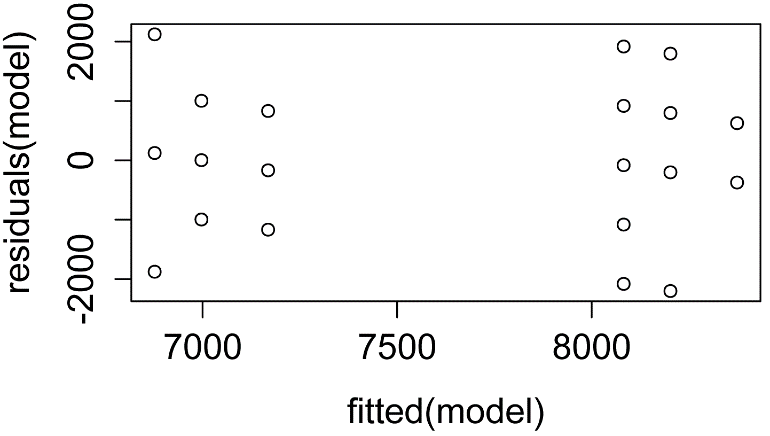##### Examples of residual plots and histograms

In the four plots below, A) Residuals.a show normally distributed and homoscedastic residuals, suggesting model assumptions were met.  B) Residuals.b show a non-normal distribution of residuals.  C)  Residuals.c show that the residuals are not independent of the fitted values.  In this case, the model needs to be modified in order to describe the data well.  D) Residuals.d show heteroscedasticity, since variability in the residuals is greater for large fitted values than for small fitted values.  (Adapted from similar plots in Tabachnick, 2001).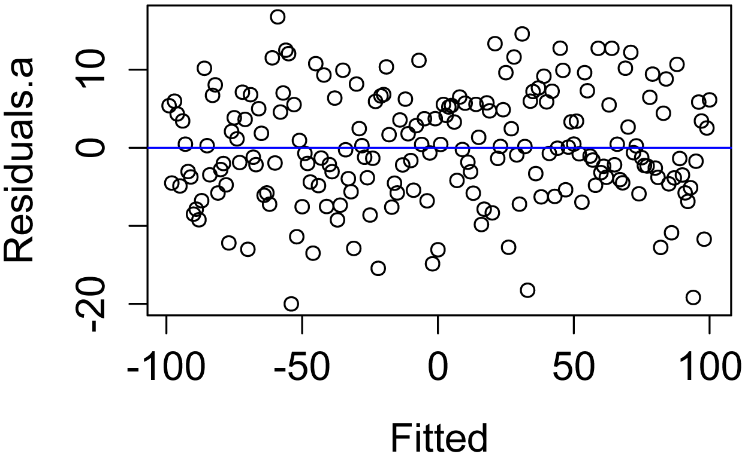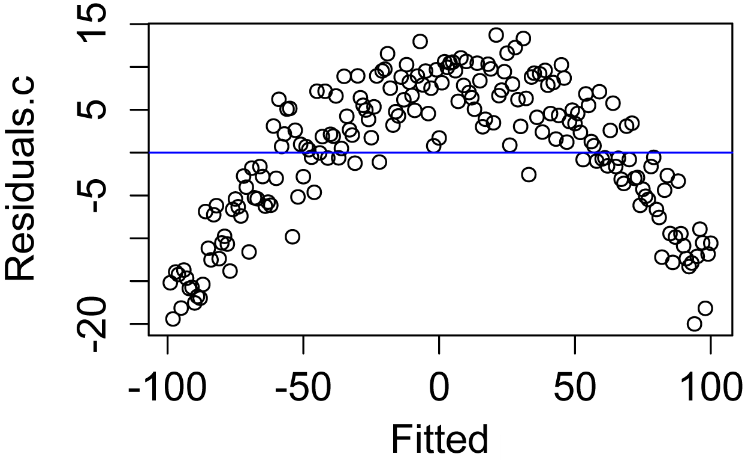The following plots are histograms of the same residuals shown in the previous plots.  A)  Residuals.a are reasonably-close to normally distributed.  B) Residuals.b are highly skewed (left, or negative).  C) Residuals.c are moderately negatively skewed.  This distribution would probably not cause too much havoc with most parametric tests, but, depending on the circumstances, I would probably try to transform a variable or find a better-fitting model.  D) Residuals.d are symmetric, but leptokurtic.  I probably wouldn’t be too concerned with the distribution.

Results from the describe function in the psych package are shown below the histograms.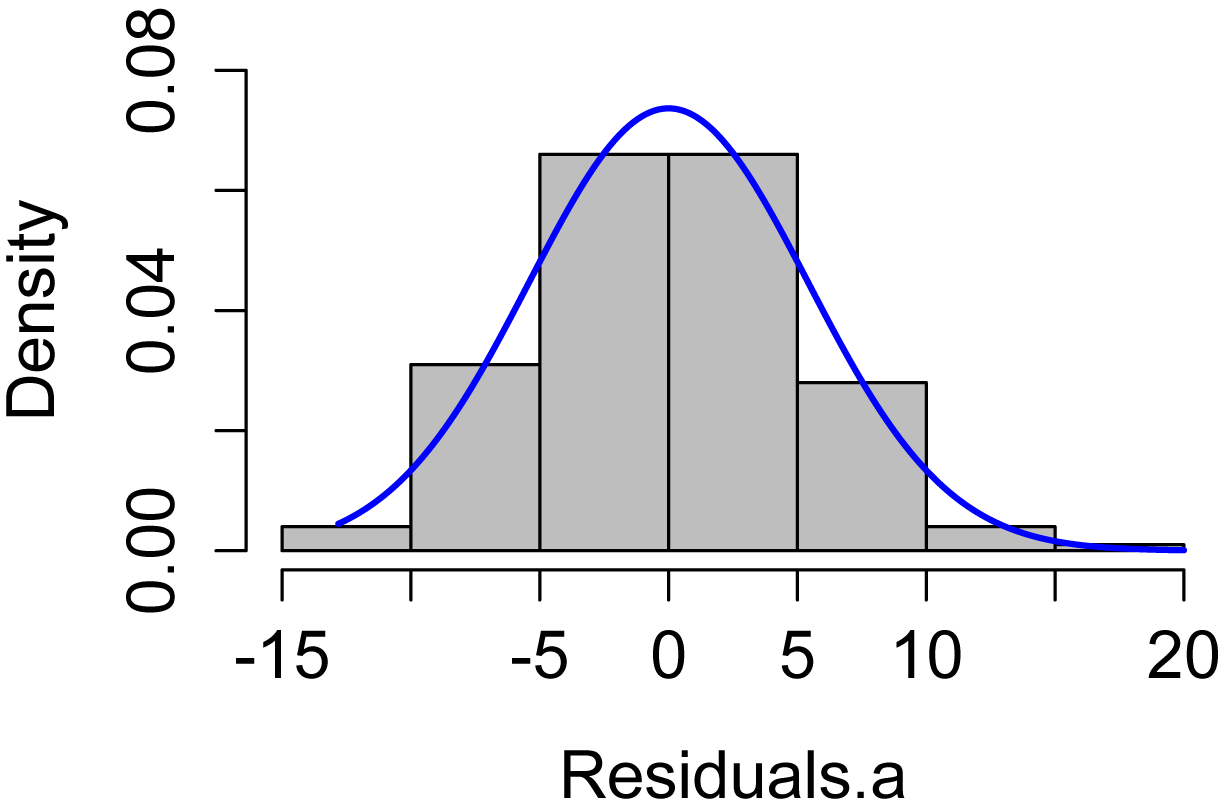### describe(Residuals.a)

vars   n mean   sd median trimmed  mad min   max range  skew kurtosis   se
1    1 200    0 6.82   0.63    0.07 6.91 -20 18.51 38.51 -0.14     0.03 0.48

### describe(Residuals.b)

vars   n mean   sd median trimmed  mad min  max range  skew kurtosis   se
1    1 200    0 3.77   1.38    0.86 1.62 -20 2.62 22.62 -2.53      7.2 0.27

### describe(Residuals.c)

vars   n mean   sd median trimmed  mad min   max range  skew kurtosis   se
1    1 200    0 6.79   1.25    0.48 7.75 -20 12.88 32.88 -0.54    -0.42 0.48

### describe(Residuals.d)

vars   n mean   sd median trimmed  mad min   max range  skew kurtosis   se
1    1 200    0 5.53   0.11    0.21 3.83 -20 16.57 36.57 -0.48     1.94 0.39

Other diagnostic plots

Using the plot function for most common types of models will show diagnostic plots or other useful plots for the model.  For linear models, the plot function produces plots of 1) residuals vs. fitted values; 2) normal quantile–quantile plot; 3) square root of standardized residuals vs. fitted values; and 4) standardized residuals vs. leverage.

While these are useful diagnostic plots, their interpretation will not be discussed here.

plot(model)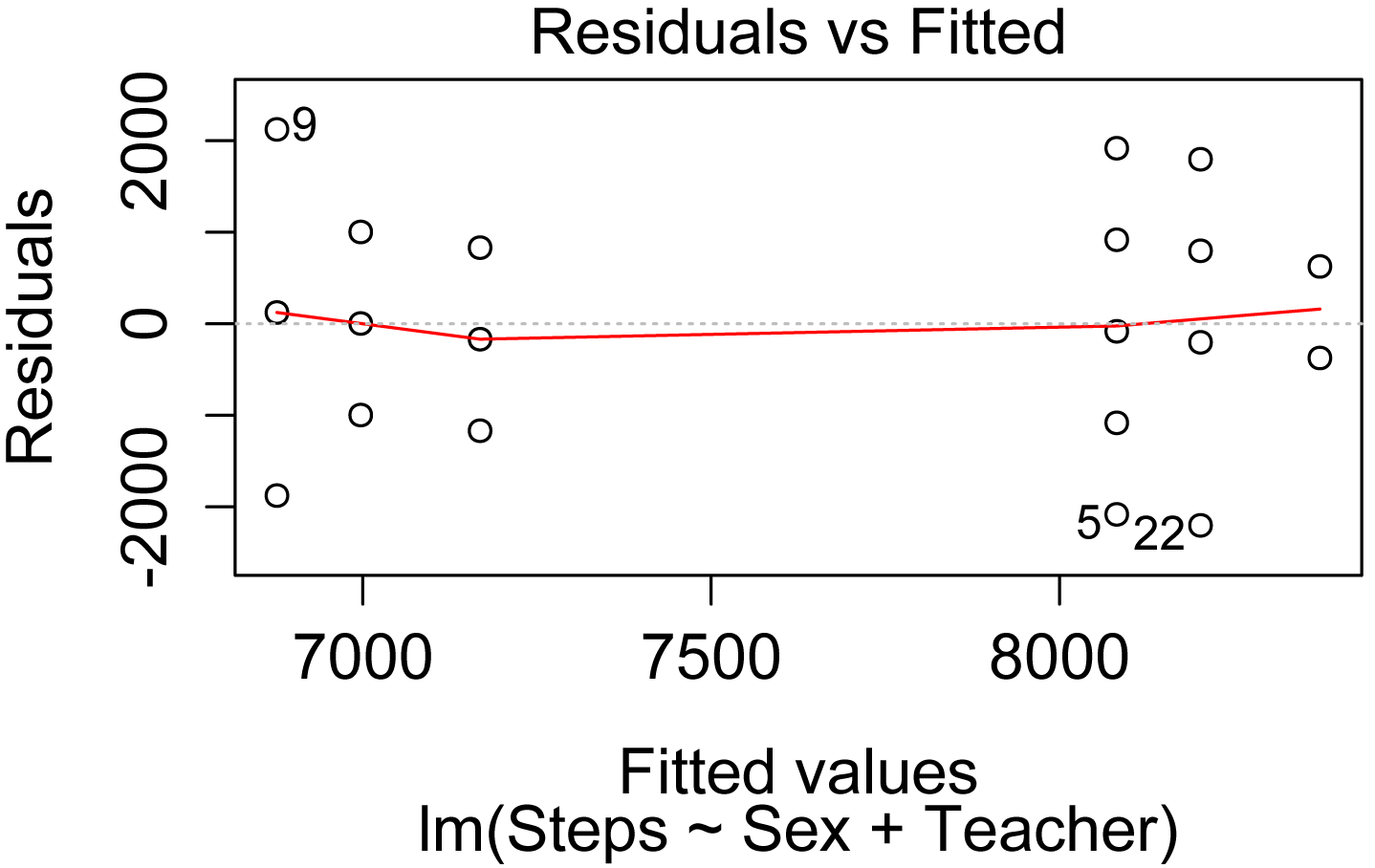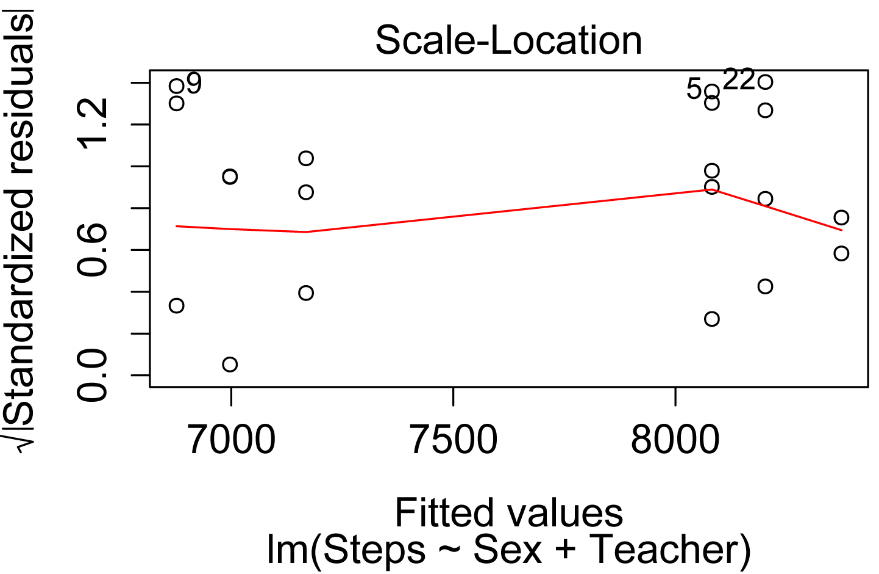#### Steps to handle violations of assumptions

If residuals show heteroscedasticity or a non-normal distribution, the analysis must be modified in some way to get valid results.  Some options include the following.

1. Select the correct parametric test and check assumptions

Problems with distribution of residuals is sometimes related to the model not describing the data well.  Adding or removing terms from the model may correct this.  As an example, imagine you had plotted bivariate data and they formed a shape like a parabola.  If you fit a straight line to this data, you might get residuals that look like Residuals.c above.  Adding an x2 term, or other term to add curvature to the fitted model, might correct this.  A similar situation can be encountered in analysis of variance and similar tests; it is sometimes helpful to add other terms or covariates.

3. Transform data and repeat your original analysis

The dependent variable, or other variables, can be transformed so that model assumptions are met.  The tests are then performed on these transformed data.  See the Transforming Data chapter for details.

4. Use nonparametric tests

Nonparametric tests include those discussed in the Traditional Nonparametric Tests section of this book and those chapters on permutation tests.  Quantile regression and generalized additive model may be options as well.

5. Use robust methods

There are other statistical methods which are robust to violations of parametric assumptions or are nonparametric.  See Mangiafico (2015a) and (2015b) in the “References” section for examples.

### Descriptive Statistics for Parametric Statistics

Descriptive statistics for interval/ratio data are discussed in the “Descriptive statistics for interval/ratio data” section in the Descriptive Statistics chapter.

Descriptive plots for interval/ratio data are discussed in the “Examples of basic plots for interval/ratio and ordinal data” section in the Basic Plots chapter.

“Normality” in McDonald, J.H. 2014. Handbook of Biological Statistics. www.biostathandbook.com/normality.html.

“Independence” in McDonald, J.H. 2014. Handbook of Biological Statistics. www.biostathandbook.com/independence.html.

“Homoscedasticity and heteroscedasticity” in McDonald, J.H. 2014. Handbook of Biological Statistics. www.biostathandbook.com/homoscedasticity.html.

### References

“One-way Analysis with Permutation Test” in Mangiafico, S.S. 2015a. An R Companion for the Handbook of Biological Statistics, version 1.09. rcompanion.org/rcompanion/d_06a.html.

“Two-way Anova with Robust Estimation” in Mangiafico, S.S. 2015b. An R Companion for the Handbook of Biological Statistics, version 1.09. rcompanion.org/rcompanion/d_08a.html.

Tabachnick, B.G. and S.F. Fidell. 2001. Using multivariate statistics. 4th Edition. Allyn and

Bacon, Boston, MA.

“Normality tests don't do what you think they do.” 2012. Fells Stats. blog.fellstat.com/?p=61.

### Optional analyses: formal tests for normality

Code for conducting formal tests of normality are included here.  I don’t recommend using them, but they may be instructive in training the eye to interpret histograms and Q-Q plots, or to respond to a less-savvy reviewer.

In each case, the null hypothesis is that the data distribution is not different from normal.  That is, a significant p-value (p < 0.05) suggests that data are not normally distributed.

As mentioned previously, a limitation to using these tests is that as the number of observations are increased, the ability of the test to return a significant p-value increases, even for small deviations from a normal distribution.

Input = ("
Student  Sex     Teacher  Steps  Rating
a        female  Catbus    8000   7
b        female  Catbus    9000  10
c        female  Catbus   10000   9
d        female  Catbus    7000   5
e        female  Catbus    6000   4
f        female  Catbus    8000   8
g        male    Catbus    7000   6
h        male    Catbus    5000   5
i        male    Catbus    9000  10
j        male    Catbus    7000   8
k        female  Satsuki   8000   7
l        female  Satsuki   9000   8
m        female  Satsuki   9000   8
n        female  Satsuki   8000   9
o        male    Satsuki   6000   5
p        male    Satsuki   8000   9
q        male    Satsuki   7000   6
r        female  Totoro   10000  10
s        female  Totoro    9000  10
t        female  Totoro    8000   8
u        female  Totoro    8000   7
v        female  Totoro    6000   7
w        male    Totoro    6000   8
x        male    Totoro    8000  10
y        male    Totoro    7000   7
z        male    Totoro    7000   7
")

###  Check the data frame

library(psych)

str(Data)

summary(Data)

### Remove unnecessary objects

rm(Input)

###### Define a linear model

model = lm(Steps ~ Sex + Teacher,
data = Data)

#### Shapiro–Wilk normality test

x = residuals(model)

shapiro.test(x)

Shapiro-Wilk normality test

W = 0.96256, p-value = 0.4444

#### Anderson-Darling normality test

if(!require(nortest)){install.packages("nortest")}

library(nortest)

x = residuals(model)

Anderson-Darling normality test

A = 0.39351, p-value = 0.3506

#### One-sample Kolmogorov-Smirnov test

x = residuals(model)

ks.test(x,
"pnorm",
mean = mean(x),
sd   = sd(x))

One-sample Kolmogorov-Smirnov test

D = 0.1399, p-value = 0.6889

#### D'Agostino Normality Test

if(!require(fBasics)){install.packages("fBasics")}

library(fBasics)

x = residuals(model)

dagoTest(x)

Title:
D'Agostino Normality Test

Test Results:
STATISTIC:
Chi2 | Omnibus: 0.1071
Z3  | Skewness: -0.3132
Z4  | Kurtosis: 0.0948
P VALUE:
Omnibus  Test: 0.9479
Skewness Test: 0.7541
Kurtosis Test: 0.9245

### Optional analyses: formal tests for homogeneity of variance

Code for formal tests of homogeneity of variance among groups is presented here.  I don’t recommend using them, but instead recommend using diagnostic plots.

In each case, the null hypothesis is that the variance among groups is not different.  That is, a significant p-value (p < 0.05) suggests that the variance among groups is different.

Input = ("
Student  Sex     Teacher  Steps  Rating
a        female  Catbus    8000   7
b        female  Catbus    9000  10
c        female  Catbus   10000   9
d        female  Catbus    7000   5
e        female  Catbus    6000   4
f        female  Catbus    8000   8
g        male    Catbus    7000   6
h        male    Catbus    5000   5
i        male    Catbus    9000  10
j        male    Catbus    7000   8
k        female  Satsuki   8000   7
l        female  Satsuki   9000   8
m        female  Satsuki   9000   8
n        female  Satsuki   8000   9
o        male    Satsuki   6000   5
p        male    Satsuki   8000   9
q        male    Satsuki   7000   6
r        female  Totoro   10000  10
s        female  Totoro    9000  10
t        female  Totoro    8000   8
u        female  Totoro    8000   7
v        female  Totoro    6000   7
w        male    Totoro    6000   8
x        male    Totoro    8000  10
y        male    Totoro    7000   7
z        male    Totoro    7000   7
")

###  Check the data frame

library(psych)

str(Data)

summary(Data)

### Remove unnecessary objects

rm(Input)

###### Define a linear model

model = lm(Steps ~ Sex + Teacher,
data = Data)

Bartlett’s test for homogeneity of variance

Bartlett’s test is known to be sensitive to non-normality in samples.  That is, non-normal samples can result in a significant test due to the non-normality.

x = residuals(model)

bartlett.test(x ~ interaction(Sex, Teacher),
data=Data)

Bartlett test of homogeneity of variances

Bartlett's K-squared = 3.7499, df = 5, p-value = 0.586

#### Levene’s test for homogeneity of variance

Levene’s test is an alternative to Bartlett’s that is supposedly less sensitive to departures from normality in the data.

if(!require(car)){install.packages("car")}

library(car)

x = residuals(model)

leveneTest(x ~ Sex * Teacher
data=Data,
center=mean)       ### Use the original Levene’s test

Levene's Test for Homogeneity of Variance (center = mean)

Df F value Pr(>F)
group  5  0.4457 0.8113
20

#### Brown–Forsythe or robust Levene’s test

The Brown–Forsythe modification of Levene’s test makes it more robust to departures in normality of the data.

if(!require(car)){install.packages("car")}

library(car)

x = residuals(model)

leveneTest(x ~ Sex * Teacher, data=Data)

Levene's Test for Homogeneity of Variance (center = median)

Df F value Pr(>F)
group  5  0.4015  0.842
20

if(!require(lawstat)){install.packages("lawstat")}

library(lawstat)

x = residuals(model)

levene.test(x, interaction(Data\$Sex, Data\$Teacher))

modified robust Brown-Forsythe Levene-type test based on the absolute deviations from the median

Test Statistic = 0.4015, p-value = 0.842

#### Fligner-Killeen test

The Fligner-Killeen test is another test for homogeneity of variances that is robust to departures in normality of the data.

x = residuals(model)

fligner.test(x ~ interaction(Sex, Teacher), data=Data)

Fligner-Killeen test of homogeneity of variances

Fligner-Killeen:med chi-squared = 2.685, df = 5, p-value = 0.7484